# 3.2 Domain and range  (Page 8/11)

 Page 8 / 11

## Verbal

Why does the domain differ for different functions?

The domain of a function depends upon what values of the independent variable make the function undefined or imaginary.

How do we determine the domain of a function defined by an equation?

Explain why the domain of $\text{\hspace{0.17em}}f\left(x\right)=\sqrt{x}\text{\hspace{0.17em}}$ is different from the domain of $\text{\hspace{0.17em}}f\left(x\right)=\sqrt[]{x}.$

There is no restriction on $\text{\hspace{0.17em}}x\text{\hspace{0.17em}}$ for $\text{\hspace{0.17em}}f\left(x\right)=\sqrt{x}\text{\hspace{0.17em}}$ because you can take the cube root of any real number. So the domain is all real numbers, $\text{\hspace{0.17em}}\left(-\infty ,\infty \right).\text{\hspace{0.17em}}$ When dealing with the set of real numbers, you cannot take the square root of negative numbers. So $\text{\hspace{0.17em}}x$ -values are restricted for $\text{\hspace{0.17em}}f\left(x\right)=\sqrt[]{x}\text{\hspace{0.17em}}$ to nonnegative numbers and the domain is $\text{\hspace{0.17em}}\left[0,\infty \right).$

When describing sets of numbers using interval notation, when do you use a parenthesis and when do you use a bracket?

How do you graph a piecewise function?

Graph each formula of the piecewise function over its corresponding domain. Use the same scale for the $\text{\hspace{0.17em}}x$ -axis and $\text{\hspace{0.17em}}y$ -axis for each graph. Indicate inclusive endpoints with a solid circle and exclusive endpoints with an open circle. Use an arrow to indicate $\text{\hspace{0.17em}}-\infty \text{\hspace{0.17em}}$ or Combine the graphs to find the graph of the piecewise function.

## Algebraic

For the following exercises, find the domain of each function using interval notation.

$f\left(x\right)=-2x\left(x-1\right)\left(x-2\right)$

$f\left(x\right)=5-2{x}^{2}$

$\left(-\infty ,\infty \right)$

$f\left(x\right)=3\sqrt{x-2}$

$f\left(x\right)=3-\sqrt{6-2x}$

$\left(-\infty ,3\right]$

$f\left(x\right)=\sqrt{4-3x}$

$\begin{array}{l}\\ f\left(x\right)=\sqrt[]{{x}^{2}+4}\end{array}$

$\left(-\infty ,\infty \right)$

$f\left(x\right)=\sqrt{1-2x}$

$f\left(x\right)=\sqrt{x-1}$

$\left(-\infty ,\infty \right)$

$f\left(x\right)=\frac{9}{x-6}$

$f\left(x\right)=\frac{3x+1}{4x+2}$

$\left(-\infty ,-\frac{1}{2}\right)\cup \left(-\frac{1}{2},\infty \right)$

$f\left(x\right)=\frac{\sqrt{x+4}}{x-4}$

$f\left(x\right)=\frac{x-3}{{x}^{2}+9x-22}$

$\left(-\infty ,-11\right)\cup \left(-11,2\right)\cup \left(2,\infty \right)$

$f\left(x\right)=\frac{1}{{x}^{2}-x-6}$

$f\left(x\right)=\frac{2{x}^{3}-250}{{x}^{2}-2x-15}$

$\left(-\infty ,-3\right)\cup \left(-3,5\right)\cup \left(5,\infty \right)$

$\frac{5}{\sqrt{x-3}}$

$\frac{2x+1}{\sqrt{5-x}}$

$\left(-\infty ,5\right)$

$f\left(x\right)=\frac{\sqrt{x-4}}{\sqrt{x-6}}$

$f\left(x\right)=\frac{\sqrt{x-6}}{\sqrt{x-4}}$

$\left[6,\infty \right)$

$f\left(x\right)=\frac{x}{x}$

$f\left(x\right)=\frac{{x}^{2}-9x}{{x}^{2}-81}$

$\left(-\infty ,-9\right)\cup \left(-9,9\right)\cup \left(9,\infty \right)$

Find the domain of the function $\text{\hspace{0.17em}}f\left(x\right)=\sqrt{2{x}^{3}-50x}\text{\hspace{0.17em}}$ by:

1. using algebra.
2. graphing the function in the radicand and determining intervals on the x -axis for which the radicand is nonnegative.

## Graphical

For the following exercises, write the domain and range of each function using interval notation.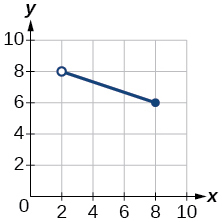domain: $\text{\hspace{0.17em}}\left(2,8\right],\text{\hspace{0.17em}}$ range $\text{\hspace{0.17em}}\left[6,8\right)\text{\hspace{0.17em}}$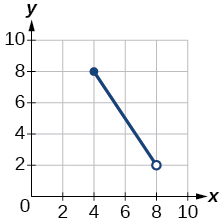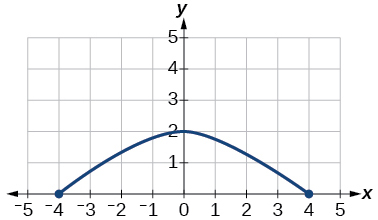domain: range: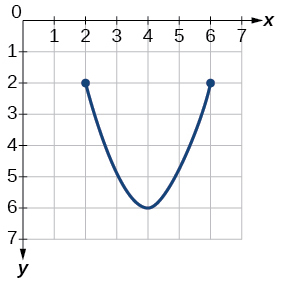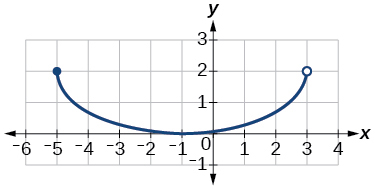domain: range: $\text{\hspace{0.17em}}\left[0,2\right]$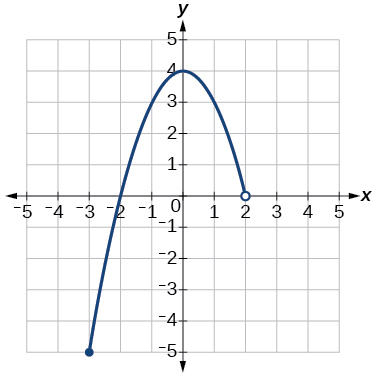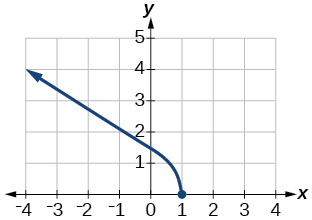domain: $\text{\hspace{0.17em}}\left(-\infty ,1\right],\text{\hspace{0.17em}}$ range: $\text{\hspace{0.17em}}\left[0,\infty \right)\text{\hspace{0.17em}}$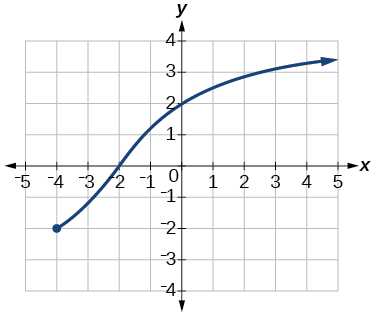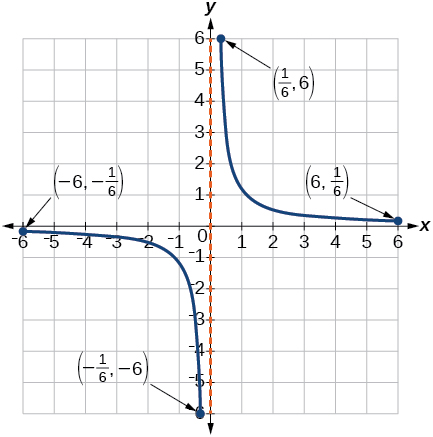domain: $\text{\hspace{0.17em}}\left[-6,-\frac{1}{6}\right]\cup \left[\frac{1}{6},6\right];\text{\hspace{0.17em}}$ range: $\text{\hspace{0.17em}}\left[-6,-\frac{1}{6}\right]\cup \left[\frac{1}{6},6\right]\text{\hspace{0.17em}}$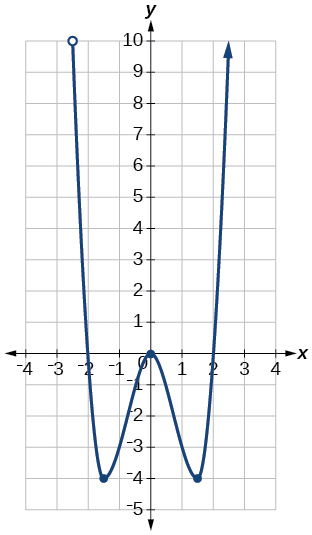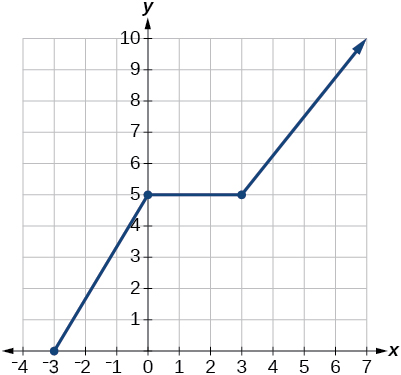domain: range: $\text{\hspace{0.17em}}\left[0,\infty \right)\text{\hspace{0.17em}}$

For the following exercises, sketch a graph of the piecewise function. Write the domain in interval notation.

$f\left(x\right)=\left\{\begin{array}{lll}x+1\hfill & \text{if}\hfill & x<-2\hfill \\ -2x-3\hfill & \text{if}\hfill & x\ge -2\hfill \end{array}$

$f\left(x\right)=\left\{\begin{array}{lll}2x-1\hfill & \text{if}\hfill & x<1\hfill \\ 1+x\hfill & \text{if}\hfill & x\ge 1\hfill \end{array}$

domain: $\text{\hspace{0.17em}}\left(-\infty ,\infty \right)$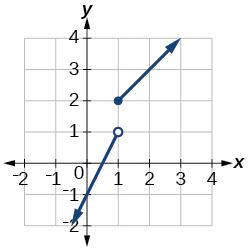$f\left(x\right)=\left\{\begin{array}{c}x+1\text{\hspace{0.17em}}\text{\hspace{0.17em}}\text{if}\text{\hspace{0.17em}}\text{\hspace{0.17em}}x<0\\ x-1\text{\hspace{0.17em}}\text{\hspace{0.17em}}\text{if}\text{\hspace{0.17em}}\text{\hspace{0.17em}}\text{\hspace{0.17em}}x>0\end{array}$

$f\left(x\right)=\left\{\begin{array}{ccc}3& \text{if}& x<0\\ \sqrt{x}& \text{if}& x\ge 0\end{array}$

domain: $\text{\hspace{0.17em}}\left(-\infty ,\infty \right)$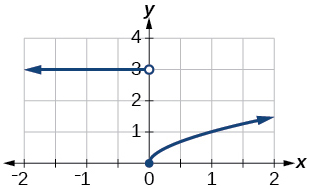$f\left(x\right)=\left\{\begin{array}{r}\hfill \begin{array}{r}\hfill {x}^{2}\\ \hfill x+2\end{array}\end{array}\text{\hspace{0.17em}}\text{\hspace{0.17em}}\begin{array}{l}\text{if}\text{\hspace{0.17em}}\text{\hspace{0.17em}}\text{\hspace{0.17em}}\text{\hspace{0.17em}}\text{\hspace{0.17em}}x<0\hfill \\ \text{if}\text{\hspace{0.17em}}\text{\hspace{0.17em}}\text{\hspace{0.17em}}\text{\hspace{0.17em}}\text{\hspace{0.17em}}x\ge 0\hfill \end{array}$

domain: $\text{\hspace{0.17em}}\left(-\infty ,\infty \right)$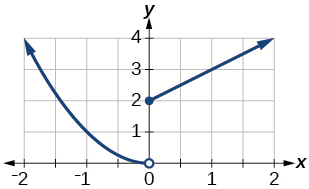$f\left(x\right)=\left\{\begin{array}{ccc}x+1& \text{if}& x<1\\ {x}^{3}& \text{if}& x\ge 1\end{array}$

$f\left(x\right)=\left\{\begin{array}{c}|x|\\ 1\end{array}\begin{array}{l}\text{\hspace{0.17em}}\text{\hspace{0.17em}}\text{\hspace{0.17em}}\text{if}\text{\hspace{0.17em}}\text{\hspace{0.17em}}\text{\hspace{0.17em}}x<2\hfill \\ \text{\hspace{0.17em}}\text{\hspace{0.17em}}\text{\hspace{0.17em}}\text{if}\text{\hspace{0.17em}}\text{\hspace{0.17em}}\text{\hspace{0.17em}}x\ge 2\hfill \end{array}$

domain: $\text{\hspace{0.17em}}\left(-\infty ,\infty \right)$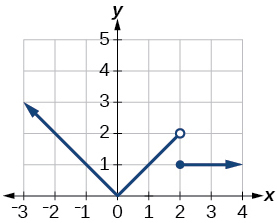## Numeric

For the following exercises, given each function $f,$ evaluate $f\left(-3\right),\text{\hspace{0.17em}}f\left(-2\right),\text{\hspace{0.17em}}f\left(-1\right),$ and $f\left(0\right).$

$f\left(x\right)=\left\{\begin{array}{lll}x+1\hfill & \text{if}\hfill & x<-2\hfill \\ -2x-3\hfill & \text{if}\hfill & x\ge -2\hfill \end{array}$

$\begin{array}{cccc}f\left(-3\right)=1;& f\left(-2\right)=0;& f\left(-1\right)=0;& f\left(0\right)=0\end{array}$

For the following exercises, given each function $\text{\hspace{0.17em}}f,\text{\hspace{0.17em}}$ evaluate $f\left(-1\right),\text{\hspace{0.17em}}f\left(0\right),\text{\hspace{0.17em}}f\left(2\right),\text{\hspace{0.17em}}$ and $\text{\hspace{0.17em}}f\left(4\right).$

$f\left(x\right)=\left\{\begin{array}{lll}7x+3\hfill & \text{if}\hfill & x<0\hfill \\ 7x+6\hfill & \text{if}\hfill & x\ge 0\hfill \end{array}$

$\begin{array}{cccc}f\left(-1\right)=-4;& f\left(0\right)=6;& f\left(2\right)=20;& f\left(4\right)=34\end{array}$

$f\left(x\right)=\left\{\begin{array}{ccc}{x}^{2}-2& \text{if}& x<2\\ 4+|x-5|& \text{if}& x\ge 2\end{array}$

$f\left(x\right)=\left\{\begin{array}{ccc}5x& \text{if}& x<0\\ 3& \text{if}& 0\le x\le 3\\ {x}^{2}& \text{if}& x>3\end{array}$

$\begin{array}{cccc}f\left(-1\right)=-5;& f\left(0\right)=3;& f\left(2\right)=3;& f\left(4\right)=16\end{array}$

For the following exercises, write the domain for the piecewise function in interval notation.

domain: $\text{\hspace{0.17em}}\left(-\infty ,1\right)\cup \left(1,\infty \right)$

$f\left(x\right)=\left\{\begin{array}{c}2x-3\\ -3{x}^{2}\end{array}\text{\hspace{0.17em}}\text{\hspace{0.17em}}\begin{array}{c}\text{if}\text{\hspace{0.17em}}\text{\hspace{0.17em}}\text{\hspace{0.17em}}x<0\\ \text{if}\text{\hspace{0.17em}}\text{\hspace{0.17em}}\text{\hspace{0.17em}}x\ge 2\end{array}$

## Technology

Graph $\text{\hspace{0.17em}}y=\frac{1}{{x}^{2}}\text{\hspace{0.17em}}$ on the viewing window $\text{\hspace{0.17em}}\left[-0.5,-0.1\right]\text{\hspace{0.17em}}$ and $\text{\hspace{0.17em}}\left[0.1,0.5\right].\text{\hspace{0.17em}}$ Determine the corresponding range for the viewing window. Show the graphs.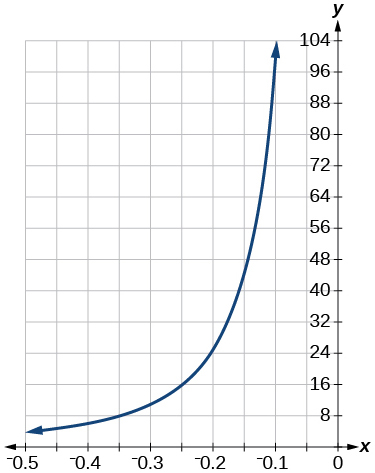window: $\text{\hspace{0.17em}}\left[-0.5,-0.1\right];\text{\hspace{0.17em}}$ range: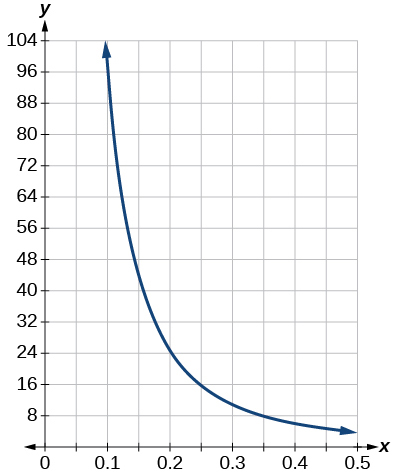window: range:

Graph $\text{\hspace{0.17em}}y=\frac{1}{x}\text{\hspace{0.17em}}$ on the viewing window $\text{\hspace{0.17em}}\left[-0.5,-0.1\right]\text{\hspace{0.17em}}$ and Determine the corresponding range for the viewing window. Show the graphs.

## Extension

Suppose the range of a function $\text{\hspace{0.17em}}f\text{\hspace{0.17em}}$ is What is the range of $\text{\hspace{0.17em}}|f\left(x\right)|?$

Create a function in which the range is all nonnegative real numbers.

Create a function in which the domain is $\text{\hspace{0.17em}}x>2.$

Many answers. One function is $\text{\hspace{0.17em}}f\left(x\right)=\frac{1}{\sqrt{x-2}}.$

## Real-world applications

The height $\text{\hspace{0.17em}}h\text{\hspace{0.17em}}$ of a projectile is a function of the time $\text{\hspace{0.17em}}t\text{\hspace{0.17em}}$ it is in the air. The height in feet for $\text{\hspace{0.17em}}t\text{\hspace{0.17em}}$ seconds is given by the function $h\left(t\right)=-16{t}^{2}+96t.$ What is the domain of the function? What does the domain mean in the context of the problem?

The domain is it takes 6 seconds for the projectile to leave the ground and return to the ground

The cost in dollars of making $\text{\hspace{0.17em}}x\text{\hspace{0.17em}}$ items is given by the function $\text{\hspace{0.17em}}C\left(x\right)=10x+500.$

1. The fixed cost is determined when zero items are produced. Find the fixed cost for this item.
2. What is the cost of making 25 items?
3. Suppose the maximum cost allowed is \$1500. What are the domain and range of the cost function, $\text{\hspace{0.17em}}C\left(x\right)?$

A laser rangefinder is locked on a comet approaching Earth. The distance g(x), in kilometers, of the comet after x days, for x in the interval 0 to 30 days, is given by g(x)=250,000csc(π30x). Graph g(x) on the interval [0, 35]. Evaluate g(5)  and interpret the information. What is the minimum distance between the comet and Earth? When does this occur? To which constant in the equation does this correspond? Find and discuss the meaning of any vertical asymptotes.
The sequence is {1,-1,1-1.....} has
how can we solve this problem
Sin(A+B) = sinBcosA+cosBsinA
Prove it
Eseka
Eseka
hi
Joel
June needs 45 gallons of punch. 2 different coolers. Bigger cooler is 5 times as large as smaller cooler. How many gallons in each cooler?
7.5 and 37.5
Nando
find the sum of 28th term of the AP 3+10+17+---------
I think you should say "28 terms" instead of "28th term"
Vedant
the 28th term is 175
Nando
192
Kenneth
if sequence sn is a such that sn>0 for all n and lim sn=0than prove that lim (s1 s2............ sn) ke hole power n =n
write down the polynomial function with root 1/3,2,-3 with solution
if A and B are subspaces of V prove that (A+B)/B=A/(A-B)
write down the value of each of the following in surd form a)cos(-65°) b)sin(-180°)c)tan(225°)d)tan(135°)
Prove that (sinA/1-cosA - 1-cosA/sinA) (cosA/1-sinA - 1-sinA/cosA) = 4
what is the answer to dividing negative index
In a triangle ABC prove that. (b+c)cosA+(c+a)cosB+(a+b)cisC=a+b+c.
give me the waec 2019 questionsByByByByByBy